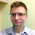## Monday, December 21, 2015

Integer operations confound middle schoolers everywhere. And understandably so. After all, imagine that everything you thought you knew about addition was suddenly turned on its head: numbers start moving the "wrong" way on the number line, adding two negatives gives you a smaller sum, and combining two integers with different signs can look a lot like what your teacher use to call subtraction.

A lot of the issue stems from students being given rules and memorizing them instead of discovering and making sense of them. Since math is the study of patterns, students need space to explore the patterns that happen when you combine positive and negative numbers.

Manipulatives like two-color counters and number lines are a pre-requisite to working with naked numbers. So after those experiences, I think Google Sheets provides some interesting possibilities.

I'm still working through the best way to do this, but a spreadsheet with pre-formatted functions seems like a good space for students to experiment with plugging in numbers, seeing the outcome, and deriving the patterns. It's sort of like a science lab for math: students input, observe, tweak, generalize, and then make meaning of their discoveries. In other words, they think like mathematicians.

Take a look at the Sheet below and let me know how it could be changed to help students understand integer addition. And if you'd like, make a copy for your Drive and mess around with it on your own.

How To Use It
1. If you're using Google Classroom, attach the Sheet to an assignment and set to "Every student gets a copy."

2. Students enter positive or negative integers in row 2, columns A and C. Anything positive will format green, negative as red to emphasize patterns. The sum will calculate automatically.Enter integers

3. Next they'll use the dropdowns in columns G-J to answer the questions about the signs of the addends. This emphasizes two things: first, if addends have the same sign, the sum will have the same sign as well. Secondly, if addends have different signs, the sum will have the sign of the number with the greater absolute value.Answer questions about equation

4. If the addends have different signs, columns K-M will be populated with the absolute value of the addends and the sum. Students need to think about how the absolute values of the addends are related to the absolute value of the sum, then select their answer from column N.

5.Absolute value relationships

6. Repeat steps 2-4 and fill in the remaining equations.

7. When students have completed the Sheet, have them discuss the patterns they observe with a partner (try these Accountable Talk posters from Education to the Core to get the conversation started).

8. Finally, have students go to the "Questions" sheet and explain their discoveries.

9.Reflection questions

Again, this is totally a work in progress and feedback would be great. I just think there's great potential in Sheets to help students experiment with math concepts and start thinking like mathematicians. It's definitely worth exploring.

1.1.